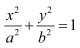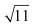• +91 9971497814
• info@interviewmaterial.com

# Chapter 14 Mathematical Reasoning Ex-14.5 Interview Questions Answers

### Related Subjects

Question 1 :
Show that the statement
p: “If x is a real number such that x3 + 4x = 0, then x is 0” is true by
(i) direct method
(iii) method of contrapositive

p:“If x is a real number such that x3 +4x = 0, then x is 0”.

Let qx isa real number such that x3 + 4x = 0

rx is0.

(i) To show that statement p istrue, we assume that q is true and then show that r istrue.

Therefore, let statement q betrue.

x3 + 4x = 0

(x2 +4) = 0

x = 0 or x+ 4 = 0

However, since isreal, it is 0.

Thus, statement r istrue.

Therefore, the given statement istrue.

(ii) To show statement p tobe true by contradiction, we assume that p is not true.

Let x be a realnumber such that x3 + 4x = 0 andlet x is not 0.

Therefore, x3 +4x = 0

x (x2 +4) = 0

x = 0or x2 + 4 = 0

x = 0or x2 = – 4

However, x isreal. Therefore, = 0, which is a contradiction since we haveassumed that x is not 0.

Thus, the given statement p istrue.

(iii) To prove statement p tobe true by contrapositive method, we assume that r is falseand prove that q must be false.

Here, r is falseimplies that it is required to consider the negation of statement r.This obtains the following statement.

rx is not 0.

It can be seen that (x2 +4) will always be positive.

x ≠ 0implies that the product of any positive real number with x isnot zero.

Let us consider the productof x with (x2 + 4).

(x2 + 4) ≠ 0

x3 + 4x ≠ 0

This shows that statement q isnot true.

Thus, it has been proved that

r  q

Therefore, the givenstatement p is true.

Question 2 :

Show that the statement “For anyreal numbers a and ba2 = b2 impliesthat a = b” is not true by giving acounter-example.

The given statement can bewritten in the form of “if-then” as follows.

If a and b arereal numbers such that a2 = b2,then a = b.

Let pa and b arereal numbers such that a2 = b2.

qa = b

The given statement has to beproved false. For this purpose, it has to be proved that if p, thenq. To showthis, two real numbers, and b, with a2 = b2 arerequired such that a ≠ b.

Let a = 1and b = –1

a2 =(1)2 = 1 and b2 = (– 1)2 =1

a2 = b2

However, a ≠ b

Thus, it can be concluded thatthe given statement is false.

Question 3 :
Show that the following statement is true by the method of contrapositive.
p: If x is an integer and x2 is even, then x is also even.

p:If x is an integer and x2 is even,then x is also even.

Let qx isan integer and x2 is even.

rx iseven.

To prove that p istrue by contrapositive method, we assume that r is false, andprove that q is also false.

Let x is noteven.

To prove that q isfalse, it has to be proved that x is not an integer or x2 isnot even.

x isnot even implies that x2 is also not even.

Therefore, statement q isfalse.

Thus, the given statement p istrue.

Question 4 :
By giving a counter example, show that the following statements are not true.
(i) p: If all the angles of a triangle are equal, then the triangle is an obtuse angled triangle.
(ii) q: The equation x2 – 1 = 0 does not have a root lying between 0 and 2.

(i) The given statement is of theform “if q then r”.

q: All theangles of a triangle are equal.

r: Thetriangle is an obtuse-angled triangle.

The given statement p hasto be proved false. For this purpose, it has to be proved that if q,then r.

To show this, angles of atriangle are required such that none of them is an obtuse angle.

It is known that the sum of allangles of a triangle is 180°. Therefore, if all the three angles are equal,then each of them is of measure 60°, which is not an obtuse angle.

In an equilateral triangle, themeasure of all angles is equal. However, the triangle is not an obtuse-angledtriangle.

Thus, it can be concluded thatthe given statement p is false.

(ii) The given statement is asfollows.

q: Theequation x2 – 1 = 0 does not have a root lyingbetween 0 and 2.

This statement has to be provedfalse. To show this, a counter example is required.

Consider x2 –1 = 0

x2 = 1

x = ±1

One root of the equation x2 –1 = 0, i.e. the root x = 1, lies between 0 and 2.

Thus, the given statement isfalse.

Question 5 :
Which of the following statements are true and which are false? In each case give a valid reason for saying so.
(i) p: Each radius of a circle is a chord of the circle.
(ii) q: The centre of a circle bisects each chord of the circle.
(iii) r: Circle is a particular case of an ellipse.
(iv) s: If x and y are integers such that x > y, then –x < –y.
(v) t:  is a rational number.

(i) The given statement p isfalse.

According to the definition ofchord, it should intersect the circle at two distinct points.

(ii) The given statement q isfalse.

If the chord is not the diameterof the circle, then the centre will not bisect that chord.

In other words, the centre of acircle only bisects the diameter, which is the chord of the circle.

(iii) The equation of an ellipseis,If we put a = b =1, then we obtain

x2 + y2 =1, which is an equation of a circle

Therefore, circle is a particularcase of an ellipse.

Thus, statement r istrue.

(iv) y

x < –y (By a rule of inequality)

Thus, the given statement s istrue.

(v) 11 is a prime number and weknow that the square root of any prime number is an irrational number.Therefore,is an irrational number.

Thus, the given statement t isfalse.

Todays Deals### Chapter 14 Mathematical Reasoning Ex-14.5 Contributorskrishan

Name:
Email:

# Latest News# 9000 interview questions in different categories